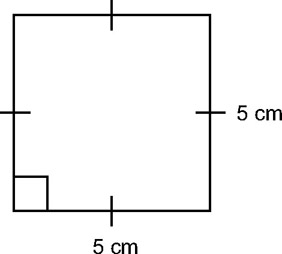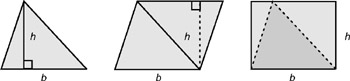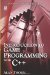# 3.6 Area and Perimeter

## 3.6 Area and Perimeter

The perimeter of a polygon is the sum of the lengths of its sides. In other words, if you took a walk along the edges of a polygon so that you walked completely around the polygon and ended up back where you started, the distance you walked would be the perimeter.

The measure of the amount of space inside the edges of any polygon is called its area, and the area of any polygon is related to the length of its sides. There are numerous ways to calculate the area of different polygons, and often the method can be to decompose the polygon into triangles, calculate the area, and then add them all up. For this reason I demonstrate how to calculate the area of a square and a triangle.

• Area of a square

The area of a square is written as A = bh (where A is area, b is base, and h is height). So, the area of the square in Figure 3.13 would be 5 × 5, meaning the area is 25 square centimeters. Because the sides of a square are all the same, the area will always be the length of one side multiplied by itself. This is why multiplying a number by itself is called squaring.Figure 3.13

• Area of a triangle

The area of a square is A = bh. Therefore, since we know a square is composed of two triangles, we know it must be A = ½ bh. In other words, (base × height) /2.Figure 3.14Introduction to Game Programming with C++ (Wordware Game Developers Library)
ISBN: 1598220322
EAN: 2147483647
Year: 2007
Pages: 225
Authors: Alan Thorn

Similar book on Amazon